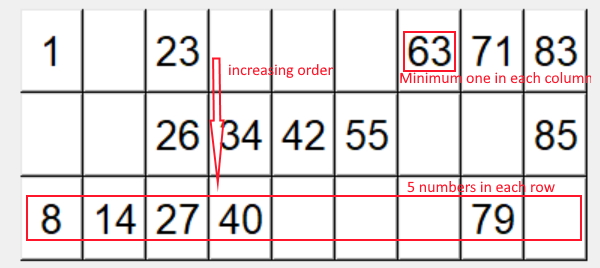# Typing Speed test

Tkinter Projects in Tkinter1. Lowest number is 1 and highest number is 90
2. Three rows and 9 columns
3. Each row will have 5 numbers
4. First column will have numbers from 1 to 10 and second column from 11 to 20 and same way other columns will have range of numbers
5. All columns will have incremental order of numbers
6. Each column should have at least one number.
7. In total there will be 15 numbers ( 5 in each row and there are three rows ).
There will be two parts for this script. First we will generate the matrix ( array ) with random number and then in second part we will add the matrix to one Tkinter window. As we will be using multiple tickets to be placed in one sheet so we will use a function my_c() to return the prepared matrix so we can call this function multiple time ( based on requirement ) to place the matrix one below the other.

Subscribe to our YouTube Channel here

## Subscribe

* indicates required
Subscribe to plus2netplus2net.com